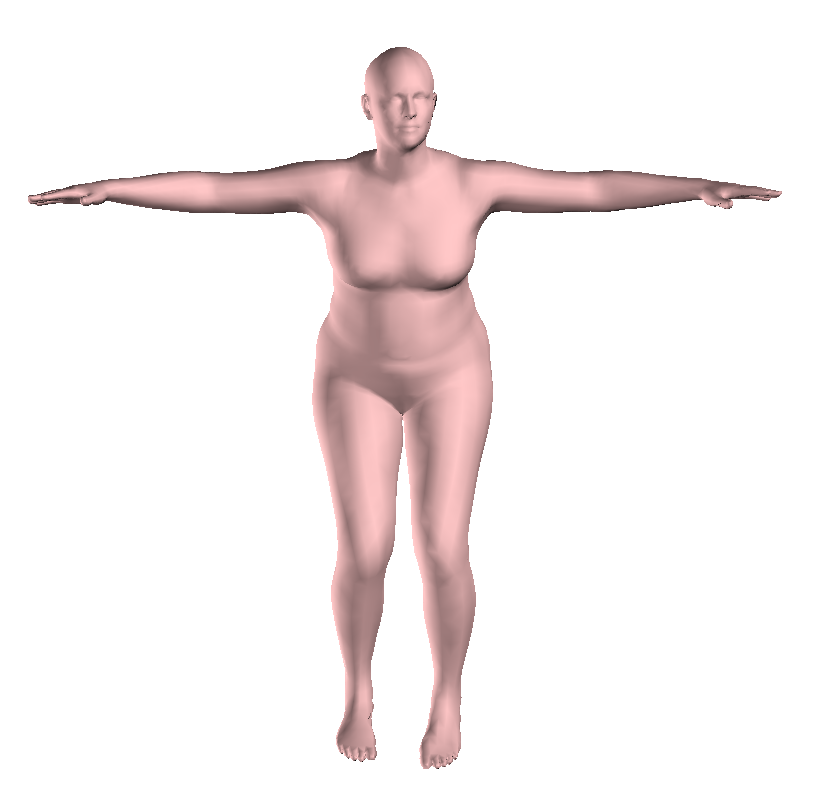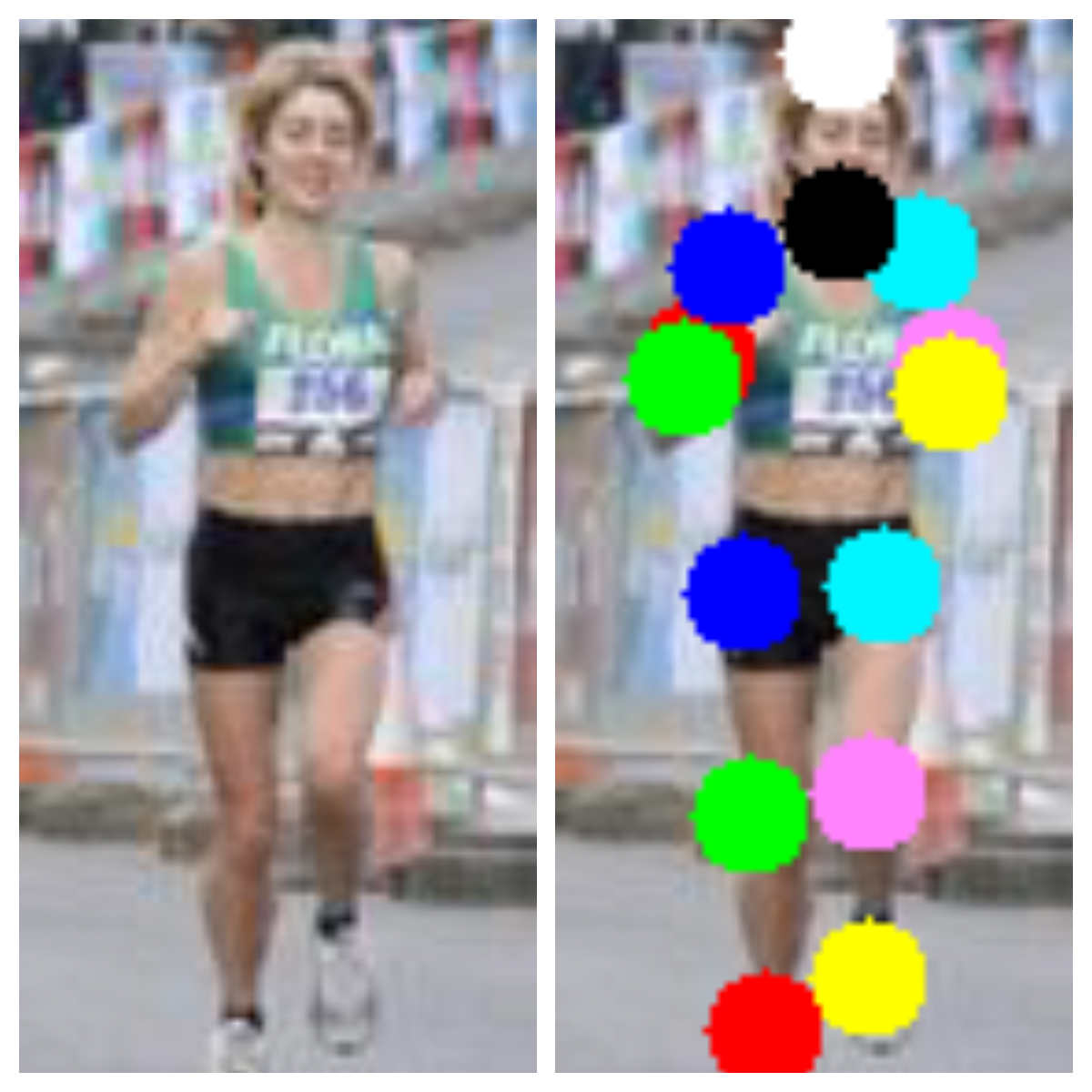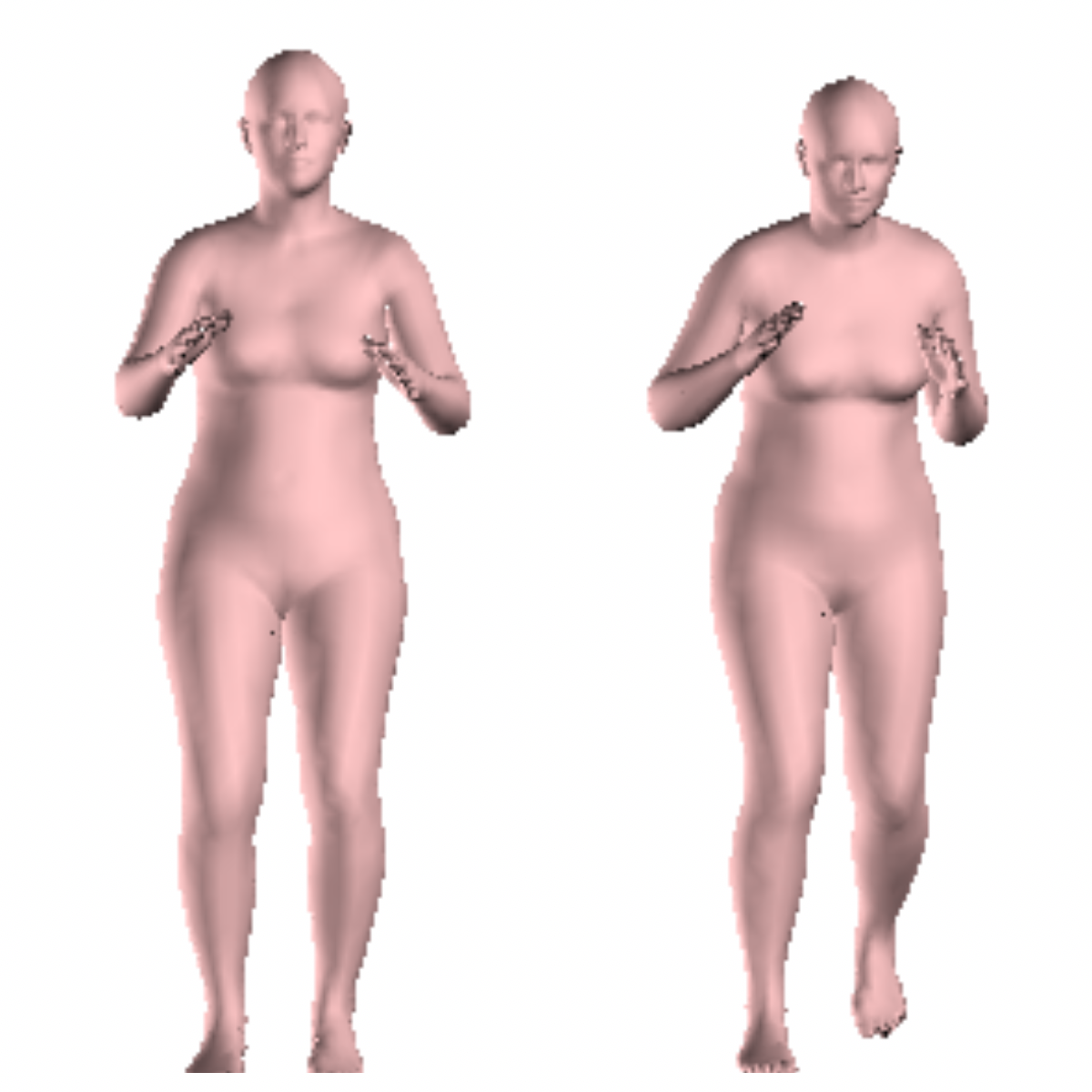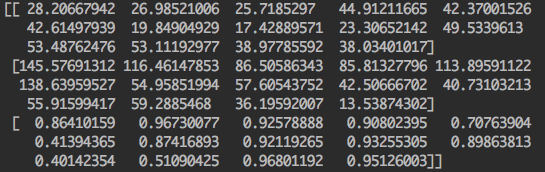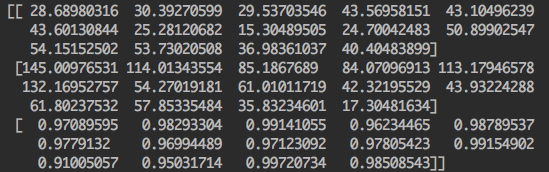## 学习报告(week 6)

### SMPLify Demo Test 2

``````with open(out_path, 'wb+') as outf:
pickle.dump(params, outf)
``````

``````with open(out_path, 'w+') as tmp:
tmp.write(params.__str__())
``````

index joint name corresponding SMPL joint ids
0 Right ankle 8
1 Right knee 5
2 Right hip 2
3 Left hip 1
4 Left knee 4
5 Left ankle 7
6 Right wrist 21
7 Right elbow 19
8 Right shoulder 17
9 Left shoulder 16
10 Left elbow 18
11 Left wrist 20
12 Neck -
13 Head top vertex 411 (see line 233:fit_3d.py)

``````The pose in 5x14 layout. The first axis is along per-joint information,
the second the joints. Information is:
1. position x,
2. position y,
3. CNN confidence,
4. CNN offset vector x,
5. CNN offset vector y.
``````

``````\$ pip install -r requirements.txt
``````

``````\$ sudo apt install caffe-cpu
``````

``````\$ pip3 install click
\$ cd models/deepercut
``````

``````\$ cd python/pose
\$ python3 ./pose_demo.py image.png --out_name=prediction --use_cpu
``````

DeepCut 的关节点将储存在 ‘prediction.npz’ 文件中。接下来，我们要运行 SMPLify 的 ‘fit_3d.py’ 来测试我们的输入图片。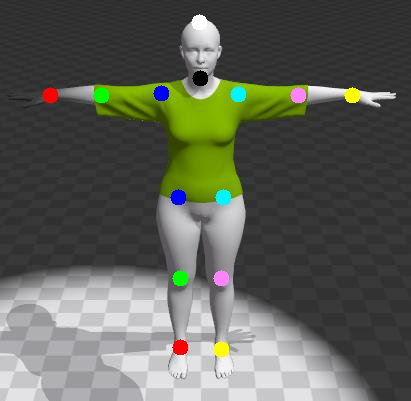``````with open(join(data_dir, 'lsp_gender.csv')) as f:
``````

``````est = np.load(join(data_dir, 'est_joints.npz'))['est_joints']
``````

``````est = np.load(join(data_dir, 'prediction.npz'))['pose']
``````

``````est = est[0:3]
est = np.reshape(est, (-1, 14, 1))
``````